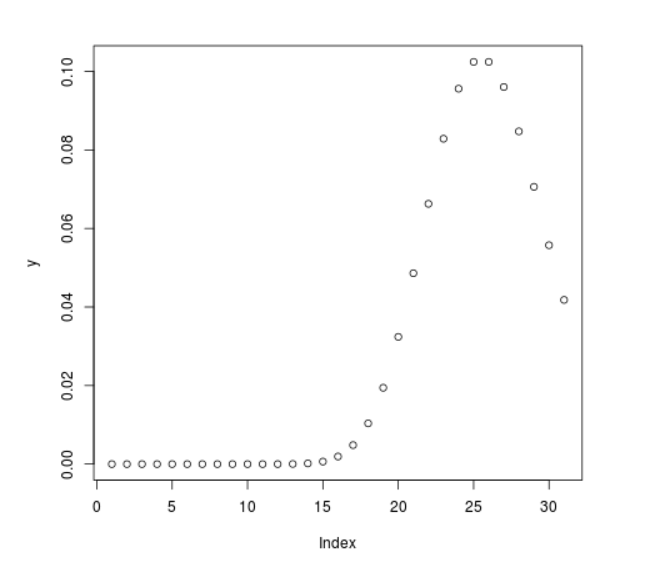# Compute the Value of Poisson Density in R Programming – dpois() Function

`dpois()` function in R Language is used to compute the Poisson Density for a set of Integer values. It also creates a desity plot of poisson distribution.

Syntax: dpois(vec, lambda)

Parameters:
vec: Sequence of integer values
lambda: Average number of events per interval

Example 1:

 `# R program to compute  ` `# Poisson Density ` ` `  `# Sequence of x-values ` `x <``-` `seq(``-``10``, ``10``, by ``=` `1``) ` ` `  `# Calling dpois() Function ` `y <``-` `dpois(x, ``lambda` `=` `5``) ` `y `

Output:

```  0.000000000 0.000000000 0.000000000 0.000000000 0.000000000 0.000000000
 0.000000000 0.000000000 0.000000000 0.000000000 0.006737947 0.033689735
 0.084224337 0.140373896 0.175467370 0.175467370 0.146222808 0.104444863
 0.065278039 0.036265577 0.018132789
```

Example 2:

 `# R program to compute  ` `# Poisson Density ` ` `  `# Sequence of x-values ` `x <``-` `seq(``-``10``, ``20``, by ``=` `1``) ` ` `  `# Calling dpois() Function ` `y <``-` `dpois(x, ``lambda` `=` `15``) ` ` `  `# Plot a graph ` `plot(y) `

Output:My Personal Notes arrow_drop_upCheck out this Author's contributed articles.

If you like GeeksforGeeks and would like to contribute, you can also write an article using contribute.geeksforgeeks.org or mail your article to contribute@geeksforgeeks.org. See your article appearing on the GeeksforGeeks main page and help other Geeks.

Please Improve this article if you find anything incorrect by clicking on the "Improve Article" button below.

Article Tags :

Be the First to upvote.

Please write to us at contribute@geeksforgeeks.org to report any issue with the above content.